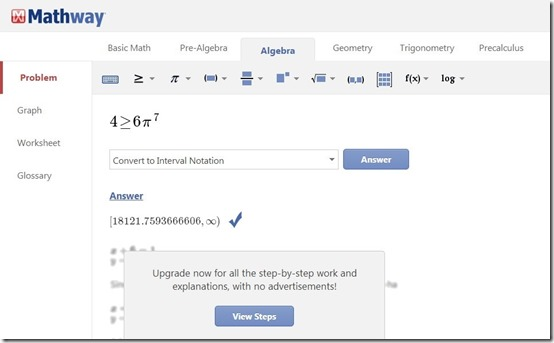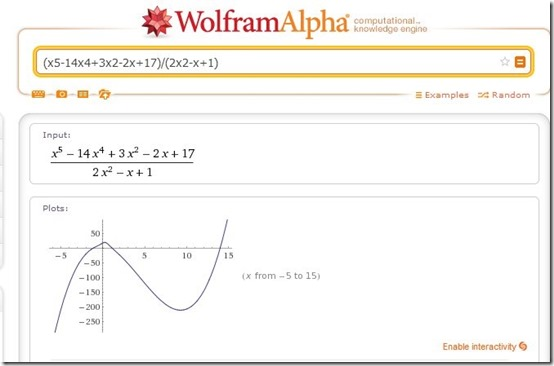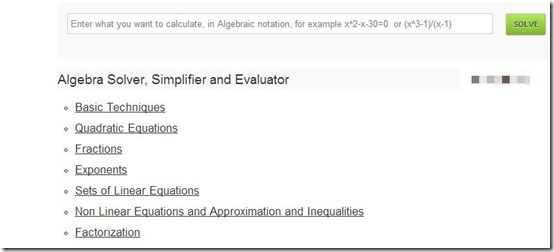Editor Ratings:
User Ratings:
[Total: 2 Average: 3]

Here is a list of 5 free websites to solve math problems online. The websites let you type all your math problems and provides step by step solutions. These websites cover all the topics of mathematics like Algebra, Statistics, Trigonometry, Geometry etc. It also covers all the levels of mathematics. From simple equations to complicated ones, it covers everything and has something for students of all age. Some of these websites also let you download worksheets.

The 5 websites to solve math problems reviewed in this article are Mathway, WolframAlpha, WebMath, Solve My Math, and Tiger Algebra.

## Mathway:The first website to solve math problems is Mathway. To use this website, you will have to create a free account first. Once, you are inside, you can start typing your problems in the provided space. The website has a very attractive interface and it supports all the mathematic fields like algebra, geometry, linear algebra, calculus, Statistics, and more. All of these options are shown on top of your screen. The symbols and signs are also provided for you, while you are typing your problem. You just have to enter the values and insert the signs or symbols you want in your problem. Step by step solution for all your problems are provided and graphs are also available. One more feature of Mathway is that you can create your worksheet. Just select the topic for your worksheet and type the number of questions you want in it. Questions will be generated randomly by the website and you can edit them, if you want.

Try Mathway here.

## WolframAlpha:Try WolframAlpha from here.

## WebMath:Third website to solve math problems is WebMath. This is a very simple to use website that has multiple calculators to solve questions ranging from General math to Trig. and Calculus. You have to select your topic from the tabs provided above, and then select a particular topic from within that. The solutions provided by the website are very descriptive and explain every step in detail. One very interesting tab on this website is “Math for everyone”. In this tab, you can make some basic math calculations like calculate compound interest, count coins (enter the number of coins and count their value in some other currency, convert temperature, speed, volume etc.

Try WebMath here.

## Solve My Math:The penultimate website to solve math problems is Solve My Math. On this website, you will find 9 different calculators for different mathematical topics. Some of the topics that this website covers are Fraction calculator, Equation calculator, Percentage calculator, Polynomial calculator etc. You have to select the calculator on the home page, and then simply solve your problem. The website leaves out some important topics like Trigonometry, Calculus. But, the solutions of the provided topics are very illustrative and in a step by step procedure. Thus, it is a pretty decent website to try out.

Try Solve My Math here.

## Tiger Algebra:The fifth and final website to solve math problems is Tiger Algebra. The only reason I have mentioned this website at the end is because it lets you solve only algebra problems. Other than that, it is a good website that covers all the regions of algebra. You can solve equations, fractions, linear algebra, non-linear algebra, and more. The problems have to be typed in the box provided above and you can get a step by step answer with just one click. Apart from the questions you type, you can also view answers for some of the recently solved problems on the website.

Try Tiger Algebra here.

You can try all these websites and solve your math problems online. They are different from the websites that teach you math lessons in the sense that you do not get to learn lessons on these websites. They are specifically meant to solve questions. Try them out and give us your feedback.

You can also read our review on 8 Free Maths Learning Websites

 Editor Ratings: User Ratings:[Total: 2 Average: 3] Free/Paid: Free Tags: mathematics